International
Tables for
Crystallography
Volume C
Mathematical, physical and chemical tables
Edited by E. Prince

International Tables for Crystallography (2006). Vol. C, ch. 4.2, pp. 191-192

Section 4.2.1.1.1. The intensity of characteristic lines

U. W. Arndta

4.2.1.1.1. The intensity of characteristic lines

| top | pdf |

The efficiency of the production of characteristic radiation has been calculated by a number of authors (see, for example, Dyson, 1973, Chap. 3). For a particular line, it depends on the fluorescence yield, that is the probability that the decay of an excited state leads to the emission of a photon, on the statistical weights of the X-ray levels involved, on the effects of the penetration and slowing down of the bombarding electrons in the target, on the fraction of electrons back-scattered out of the target, and on the contribution caused by fluorescent X-rays produced indirectly by the continuous spectrum. The emerging X-ray intensity is further affected by the partial absorption of the generated X-rays in the target.

Dyson (1973) has also reviewed calculations and measurements made of the relative intensities of different lines in the K spectrum. The ratio of theto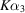intensities is very close to 0.5 for Z between 23 and 48. The ratio of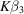torises fairly linearly with Z from 0.2 at Z = 20 to 0.4 at Z = 80 and that of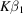tois near zero at Z = 29 and rises linearly with Z to about 0.1 at Z = 80. Relative intensities of lines in the L spectrum are given by Goldberg (1961).

Green & Cosslett (1968) have made extensive measurements of the efficiency of the production of characteristic radiation for a number of targets and for a range of electron accelerating voltages. Their results can be expressed empirically in the form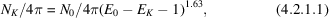where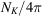is the generated number of Kα photons per steradian per incident electron, N0 is a function of the atomic number of the target, E0 is the electron energy in keV and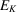is the excitation potential in keV. It should be noted thatdecreases with increasing Z.

For a copper target, this expression becomes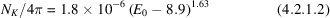or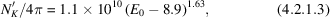where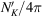is the number of Kα photons per steradian per second per milliampere of tube current.

These expressions are probably accurate to within a factor of 2 up to values of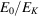of about 10. Guo & Wu (1985) found a linear relationship for the emerging number of photons with electron energy in the range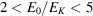.

To obtain the number of photons that emerge from the target, the above expressions have to be corrected for absorption of the generated radiation in the target. The number of photons emerging at an angleto the surface, for normal electron incidence, is usually written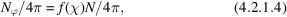where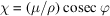(Castaing & Descamps, 1955). Green (1963) gives experimental values of the correction factor f(χ) for a series of targets over a range of electron energies. His curves for a copper target are given in Fig. 4.2.1.1. It will be noticed that the correction factor increases with increasing electron energy since the effective depth of X-ray generation increases with voltage. As a result, curves ofas a function of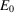have a broad maximum that is displaced towards lower voltages asdecreases, as shown in the experimental curves for copper K radiation due to Metchnik & Tomlin (1963) (Fig. 4.2.1.2). For very small take-off angles, therefore, X-ray tubes should be operated at lower than customary voltages. Note that the values in Fig. 4.2.1.2agree to within ∼40% with those of Green & Cosslett. f(χ) at constantincreases with increasing Z, thus partly compensating for the decrease in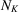, especially at small values of. A recent re-examination of the characteristic X-ray flux from Cr, Cu, Mo, Ag and W targets has been carried out by Honkimaki, Sleight & Suortti (1990).Figure 4.2.1.1 | top | pdf |f(χ) curves for Cu K-L3 at a series of different accelerating voltages (in kV). From Green (1963).Figure 4.2.1.2 | top | pdf |Experimental measurements offor Cu K-L3 as functions of the accelerating voltage for different take-off angles. From Metchnik & Tomlin (1963).

References

Castaing, R. & Descamps, J. (1955). Sur les bases physiques de l'analyse ponctuelle par spectrographie X. J. Phys. Radium, 16, 304–317.
Dyson, N. A. (1973). X-rays in atomic and nuclear physics. London: Longman.
Goldberg, M. (1961). Intensités relatives des raies X du spectre L excité par bombardement électronique des éléments lourds. J. Phys. Radium, 22, 743–748.
Green, M. (1963). The target absorption correction in X-ray microanalysis. X-ray optics and X-ray microanalysis, edited by H. Pattee, V. E. Cosslett & A. Engstrom, pp. 361–377. London: Academic Press.
Green, M. & Cosslett, V. E. (1968). Measurement of K, L and M shell X-ray production efficiencies. Br. J. Appl. Phys. Ser. 2, 1, 425–436.
Guo, C.-L. & Wu, Y.-Q. (1985). Empirical relationship between the characteristic X-ray intensity and the incident electron energy. Kexue Tongbao, 30, 1621–1627.
Honkimaki, V., Sleight, J. & Suortti, P. (1990). Characteristic X-ray flux from sealed Cr, Cu, Mo, Ag and W tubes. J. Appl. Cryst. 23, 412–417.
Metchnik, V. & Tomlin, S. G. (1963). On the absolute intensity of emission of characteristic X radiation. Proc. Phys. Soc. London, 81, 956–964.